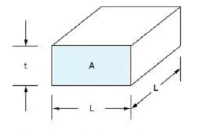[email protected] +86-755-33583558 Ext 603CONTACT
Tel: +86-755-33583558 Ext 603
Add: 407, Kanglan Fortune Center, Fuzhou Avenue, Fuyong Street, Baoan District, Shenzhen, Guangdong 518103, China

A fast estimation method of PCB wiring resistance: block statistics.

We usually need to quickly estimate the resistance value of a wire or a plane on the printed circuit board, rather than carry out tedious calculation.

Although there are available PCB layout and signal integrity calculation programs, which can accurately calculate the resistance of the wiring, we sometimes want to take a quick and rough estimation in the design process.

There is an easy way to accomplish this task, called "square statistics". With this method, the resistance of any geometry can be accurately estimated in a few seconds (the accuracy is about 10%).

Once the method is mastered, the PCB area to be estimated can be divided into several blocks. After counting the number of all blocks, the resistance value of the whole wiring or plane can be estimated.

Basic concepts

The key concept of block statistics is that the resistance value of any size square PCB is the same as that of other sizes.

The resistance of a square depends only on the resistivity and thickness of the conductive material. This concept can be applied to any type of conductive material.

The volume resistivity of copper is 0.67 μ Ω / in.

Let's start with the copper block in Figure 1. The length of the copper block is l, the width is l (because it is a square), the thickness is t, and the cross-sectional area of the copper foil area through which the current passes is a.

The resistance of the copper block can be simply expressed as R = ρ L / A, where ρ is the resistivity of copper (which is the inherent characteristic of the material, and is 0.67 μ Ω / in at 25 ℃).Note, however, that section a is the product of length L and thickness t (a = LT). L in the denominator and l in the molecule cancel each other, leaving only r = ρ / T.

Therefore, the resistance of copper block has nothing to do with the size of the block, it only depends on the resistivity and thickness of the material.

If we know the resistance value of copper blocks of any size, and can decompose the whole line to be estimated into several blocks, we can add (count) the number of blocks, and get the total resistance of the line.

The above is a simple method to estimate the DC resistance of printed circuit board wiring or plane recommended by PCB manufacturing.

Related Articles
• TEL:+86-755-33583558 Ext 603
• EMAIL:[email protected]
• ADDRESS:407, Kanglan Fortune Center, Fuzhou Avenue, Fuyong Street, Baoan District, Shenzhen, Guangdong 518103, China